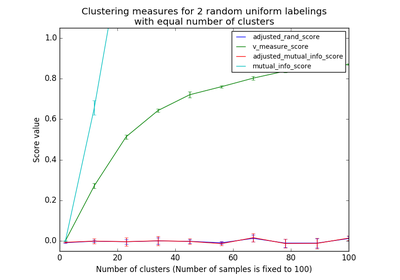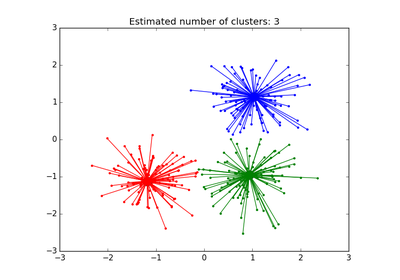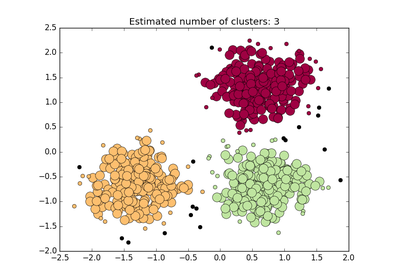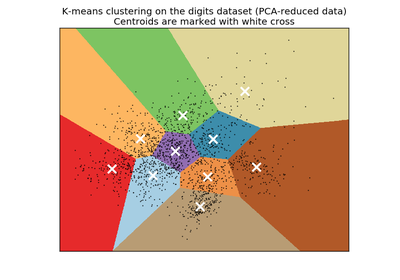# `sklearn.metrics`.v_measure_score¶

`sklearn.metrics.``v_measure_score`(labels_true, labels_pred)[source]

V-measure cluster labeling given a ground truth.

This score is identical to `normalized_mutual_info_score`.

The V-measure is the harmonic mean between homogeneity and completeness:

```v = 2 * (homogeneity * completeness) / (homogeneity + completeness)
```

This metric is independent of the absolute values of the labels: a permutation of the class or cluster label values won’t change the score value in any way.

This metric is furthermore symmetric: switching `label_true` with `label_pred` will return the same score value. This can be useful to measure the agreement of two independent label assignments strategies on the same dataset when the real ground truth is not known.

Read more in the User Guide.

Parameters: labels_true : int array, shape = [n_samples] ground truth class labels to be used as a reference labels_pred : array, shape = [n_samples] cluster labels to evaluate v_measure : float score between 0.0 and 1.0. 1.0 stands for perfectly complete labeling

References

Examples

Perfect labelings are both homogeneous and complete, hence have score 1.0:

```>>> from sklearn.metrics.cluster import v_measure_score
>>> v_measure_score([0, 0, 1, 1], [0, 0, 1, 1])
1.0
>>> v_measure_score([0, 0, 1, 1], [1, 1, 0, 0])
1.0
```

Labelings that assign all classes members to the same clusters are complete be not homogeneous, hence penalized:

```>>> print("%.6f" % v_measure_score([0, 0, 1, 2], [0, 0, 1, 1]))
...
0.8...
>>> print("%.6f" % v_measure_score([0, 1, 2, 3], [0, 0, 1, 1]))
...
0.66...
```

Labelings that have pure clusters with members coming from the same classes are homogeneous but un-necessary splits harms completeness and thus penalize V-measure as well:

```>>> print("%.6f" % v_measure_score([0, 0, 1, 1], [0, 0, 1, 2]))
...
0.8...
>>> print("%.6f" % v_measure_score([0, 0, 1, 1], [0, 1, 2, 3]))
...
0.66...
```

If classes members are completely split across different clusters, the assignment is totally incomplete, hence the V-Measure is null:

```>>> print("%.6f" % v_measure_score([0, 0, 0, 0], [0, 1, 2, 3]))
...
0.0...
```

Clusters that include samples from totally different classes totally destroy the homogeneity of the labeling, hence:

```>>> print("%.6f" % v_measure_score([0, 0, 1, 1], [0, 0, 0, 0]))
...
0.0...
```

## Examples using `sklearn.metrics.v_measure_score`¶Biclustering documents with the Spectral Co-clustering algorithmAdjustment for chance in clustering performance evaluationDemo of affinity propagation clustering algorithmDemo of DBSCAN clustering algorithmA demo of K-Means clustering on the handwritten digits dataClustering text documents using k-means